Courses

# Test: Turbulent Flow - 2

## 9 Questions MCQ Test Fluid Mechanics | Test: Turbulent Flow - 2

Description
This mock test of Test: Turbulent Flow - 2 for Mechanical Engineering helps you for every Mechanical Engineering entrance exam. This contains 9 Multiple Choice Questions for Mechanical Engineering Test: Turbulent Flow - 2 (mcq) to study with solutions a complete question bank. The solved questions answers in this Test: Turbulent Flow - 2 quiz give you a good mix of easy questions and tough questions. Mechanical Engineering students definitely take this Test: Turbulent Flow - 2 exercise for a better result in the exam. You can find other Test: Turbulent Flow - 2 extra questions, long questions & short questions for Mechanical Engineering on EduRev as well by searching above.
QUESTION: 1

### In a turbulent flow in a pipe, the shear stress is

Solution: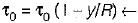shear stress variation where y is distance from pipe wall, so, the shear stress varies linearly with the distance from the boundary to become zero at the center.

QUESTION: 2

### Water of kinematic viscosity v = 1 centistoke flows through a 10 mm diameter pipe. The critical flow in this pipe would correspond to a discharge of approximately

Solution: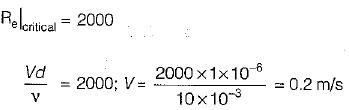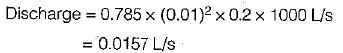QUESTION: 3

### Shear velocity is

Solution: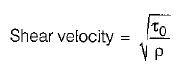QUESTION: 4

Friction factor f in laminar and turbulent flow in a pipe varies as Re-1 and Re-0.25 respectively. If V\s the average velocity, the pressure drop in a horizontal pipe for laminar and turbulent flow respectively will be proportional to

Solution: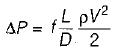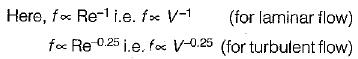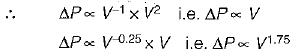QUESTION: 5

For hydrodynamically smooth pipes, the friction factor f

Solution:
QUESTION: 6

The critical value of reynolds number for transition from laminar to turbulent boundary layer in external flows is taken as

Solution:

Critical Reynolds number for external flow
(Re)Cr = 5 x 105

QUESTION: 7

The logarithmic velocity distributions observed in

Solution:

Logarithmic profile exist is in turbulent flow.

QUESTION: 8

In a fully turbulent flow through a rough pipe, the friction factor ‘f’ is (Re is Reynolds number and ξs/D is relative roughness)

Solution:
QUESTION: 9

Using the Prandtl's mixing length concept, how is the turbulent shear stress expressed?

Solution:

Turbulent shear stress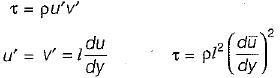(Smarter way to solve this kind of question is to check for units)